# Numpy array shape: The Complete Guide

NumPy array has attributes like the shape of the array and the dimension of the array. It has attributes that modify the array size. It resizes the array. We can also find the bytes of the items.

NumPy provides a high-performance multidimensional array and the necessary tools to calculate with and manipulate these arrays.

SciPy builds on this and offers many methods that operate on numpy arrays and are helpful for different types of scientific and engineering applications.

## np.shape

To find the shape of a numpy array in Python, use the ndarray.shape() attribute. The shape array attribute returns the tuple consisting of array dimensions.

It can also be used to resize the array. Let’s see the example in Jupyter Notebook.

Write the following code inside the cell to import the NumPy library.

`import numpy as np`

Then we need to create a NumPy array from Python List and output its shape. See the below code.

```app_list = [18, 0, 21, 30, 46]
np_app_list = np.array(app_list)
np_app_list.shape```

Here, we have defined one list, converted it into the numpy array, and then figured out print its shape. See the below output. You can run the cell using Ctrl + Enter key.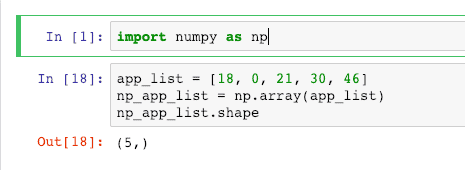The above-defined array is a single-dimension array. We can define the multidimensional array using the following code.

```app_list = [[18, 0, 21], [30, 46, 21], [19, 21, 18]]
np_app_list = np.array(app_list)
np_app_list.shape```

Here, we have defined the 3*3 dimensional Array.

See the output.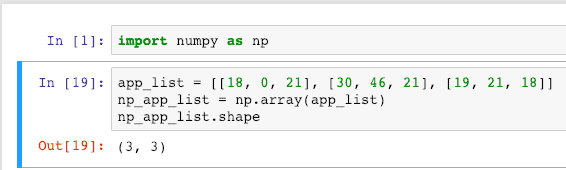## NumPy Shape To Resize Array

The following example shows how we can use the NumPy shape attribute to resize the array.

```app_list = [[18, 0, 21], [30, 46, 21]]
np_app_list = np.array(app_list)
np_app_list.shape = (3, 2)
np_app_list```

Now, we have defined a 2*3 array and changed its shape to a 3*2 array.

See the output below.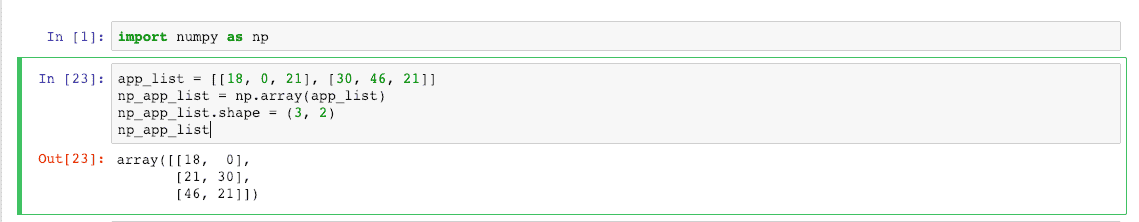We have used the shape attribute to change the shape of the array, which means it will turn rows into columns and columns into rows.

## numpy.itemsize

The itemsize attribute returns the length of each element of an array in bytes. See the below example.

```np_app_list = np.array([18, 0, 21], dtype=np.int8)
np_app_list.itemsize```

Here, when creating a numpy array, we have passed the second argument, dtype, which means the items datatype, and it is int8. That means the bytes of each character is 1.

See the below output.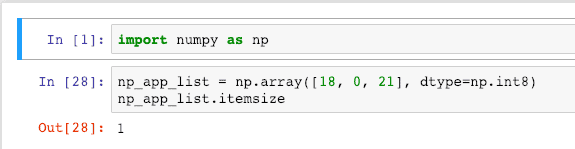If we take float32, it will return the in the output.

## numpy.ndim

The ndim attribute returns the dimension of a numpy array.

```npdata = np.array([[21, 19], [18, 21]])
npdata.ndim```

See the output.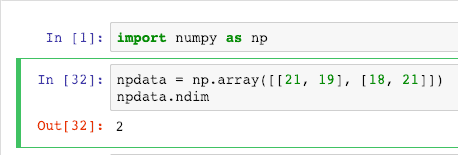That’s it.

This site uses Akismet to reduce spam. Learn how your comment data is processed.